# Some Examples of Cumulative Mean with {TidyDensity}

rtip
tidydensity
Author

Steven P. Sanderson II, MPH

Published

March 22, 2023

# Introduction

Cumulative mean is a statistical measure that calculates the mean of a set of numbers up to a certain point in time or after a certain number of observations. It is also known as a running average or moving average.

Cumulative mean can be useful in a variety of contexts. For example:

• Tracking progress: Cumulative mean can be used to track progress over time. For instance, a teacher might use it to track the average test scores of her students throughout the school year.

• Analyzing trends: Cumulative mean can help identify trends in data. For example, a business might use it to track the average revenue generated by a new product over the course of several months.

• Smoothing data: Cumulative mean can be used to smooth out fluctuations in data. For instance, a meteorologist might use it to calculate the average temperature over the course of a year, which would help to smooth out the effects of daily temperature fluctuations.

In summary, cumulative mean is a useful statistical measure that can help track progress, analyze trends, and smooth out fluctuations in data.

# Function

The function we will review is `cmean()` from the `{TidyDensity}` R package. Let’s take a look at it.

``cmean()``

The only argument is `.x` which is a numeric vector as this is a vectorized function. Let’s see it in use.

# Example

``library(TidyDensity)``

Ok now let’s make some data. For this we are going to use the simple `rnorm()` function.

``````x <- rnorm(100)

`` -0.8293250 -1.2983499  2.2782337 -0.1521549  0.6859169  0.3809020``

Ok, now that we have our vector, let’s run it through the function and see what it outputs and then we will graph it.

``````cmx <- cmean(x)
`````` -0.8293249774 -1.0638374319  0.0501862766 -0.0003990095  0.1368641726
  0.1775371452``````

Now let’s graph it.

``plot(cmx, type = "l")``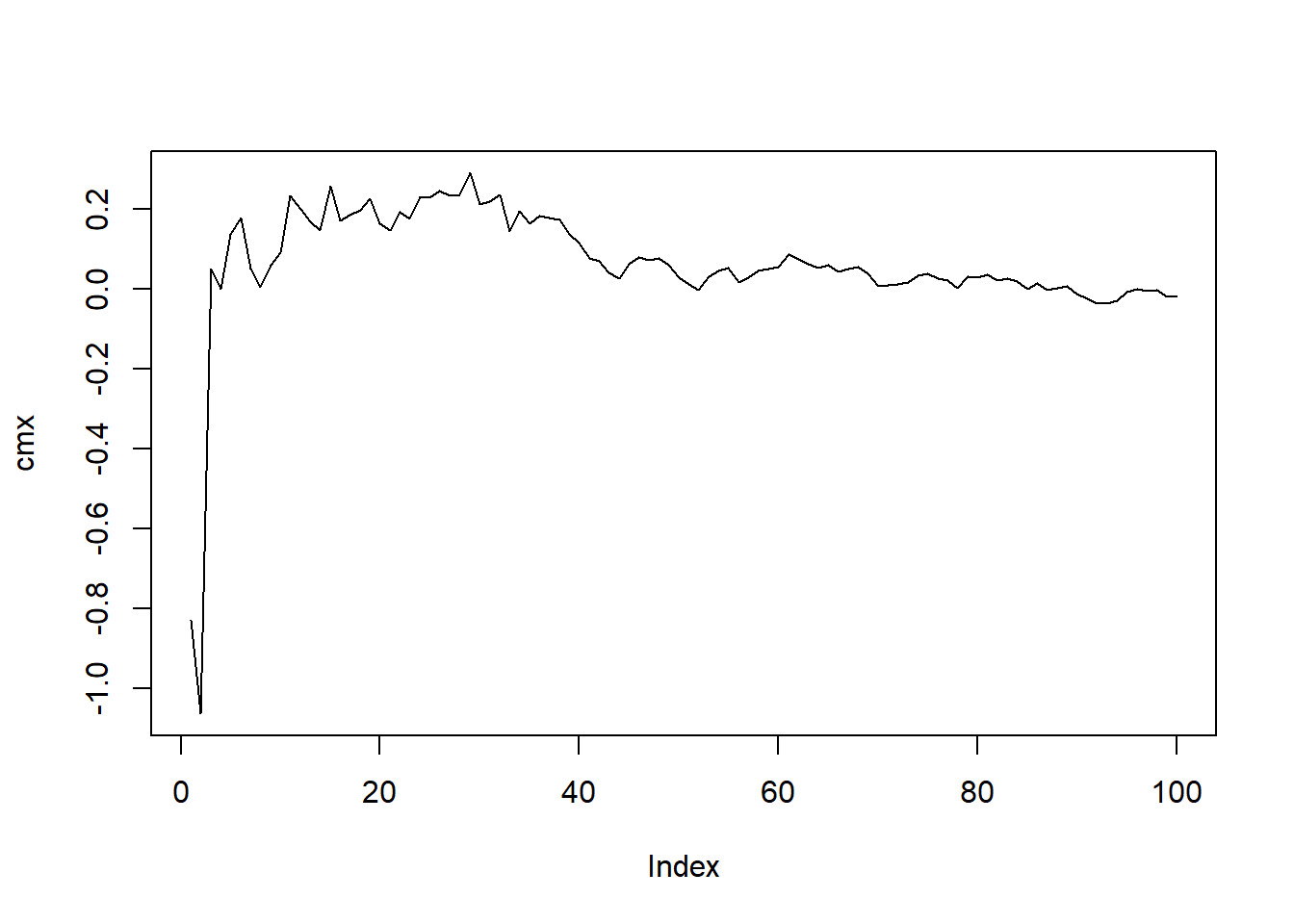Ok nice, so can we do this on grouped data or lists of data? Of course! First let’s use a for loop to generate a list of rnorm() values.

``````# Initialize an empty list to store the generated values
my_list <- list()

# Generate values using rnorm(5) in a for loop and store them in the list
for (i in 1:5) {
my_list[[i]] <- rnorm(100)
}

# Print the generated list
``````[]
 -0.8054353 -0.4596541 -0.2362475  1.1486398 -0.7242154  0.5184610

[]
  0.3243327  0.7170802 -0.5963424 -1.0307104  0.3388504  0.5717486

[]
  1.7360816 -1.0359467 -0.3206138 -1.2157684 -0.8841356  0.1856481

[]
 -1.1401642 -0.4437817 -0.2555245 -0.1809040 -0.2131763 -0.1251750

[]
  0.08835903 -1.79153379 -2.15010900  0.67344844  1.06125849  0.99848796``````

Now that we have our list object let’s go ahead and plot the values out after we pass the data through `cmean()`.

``````library(purrr)

my_list |>
map(\(x) x |> cmean() |> plot(type = "l"))``````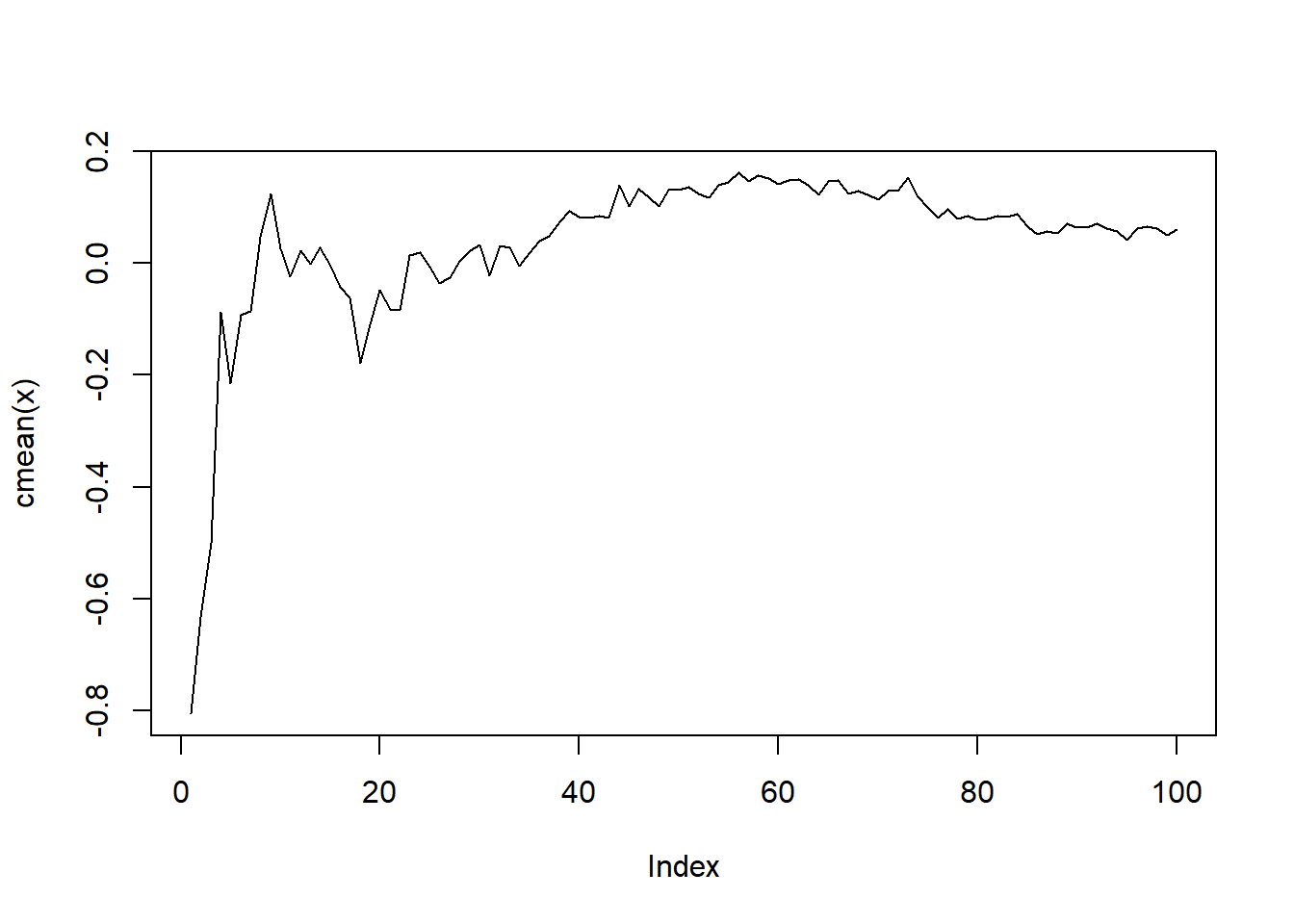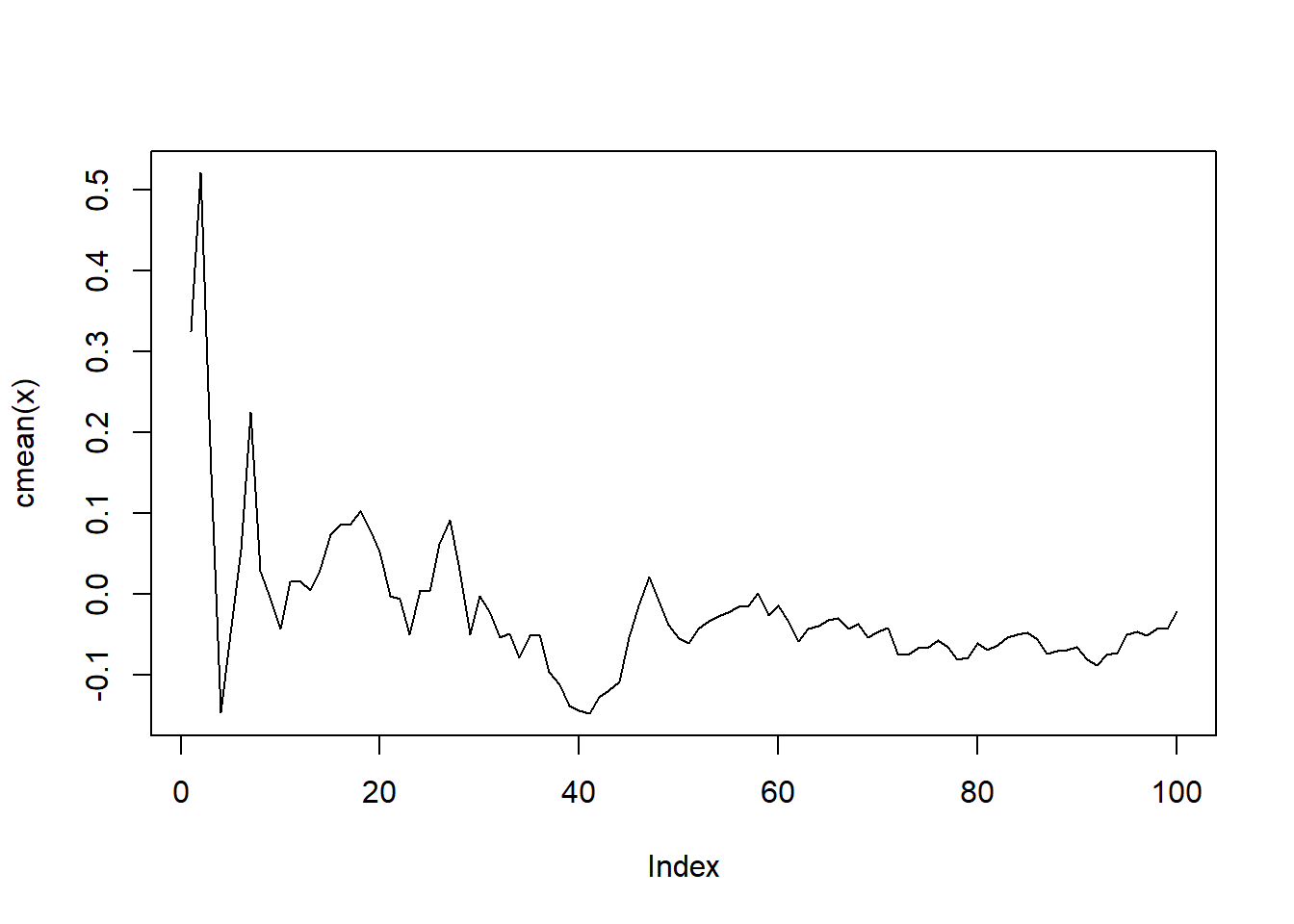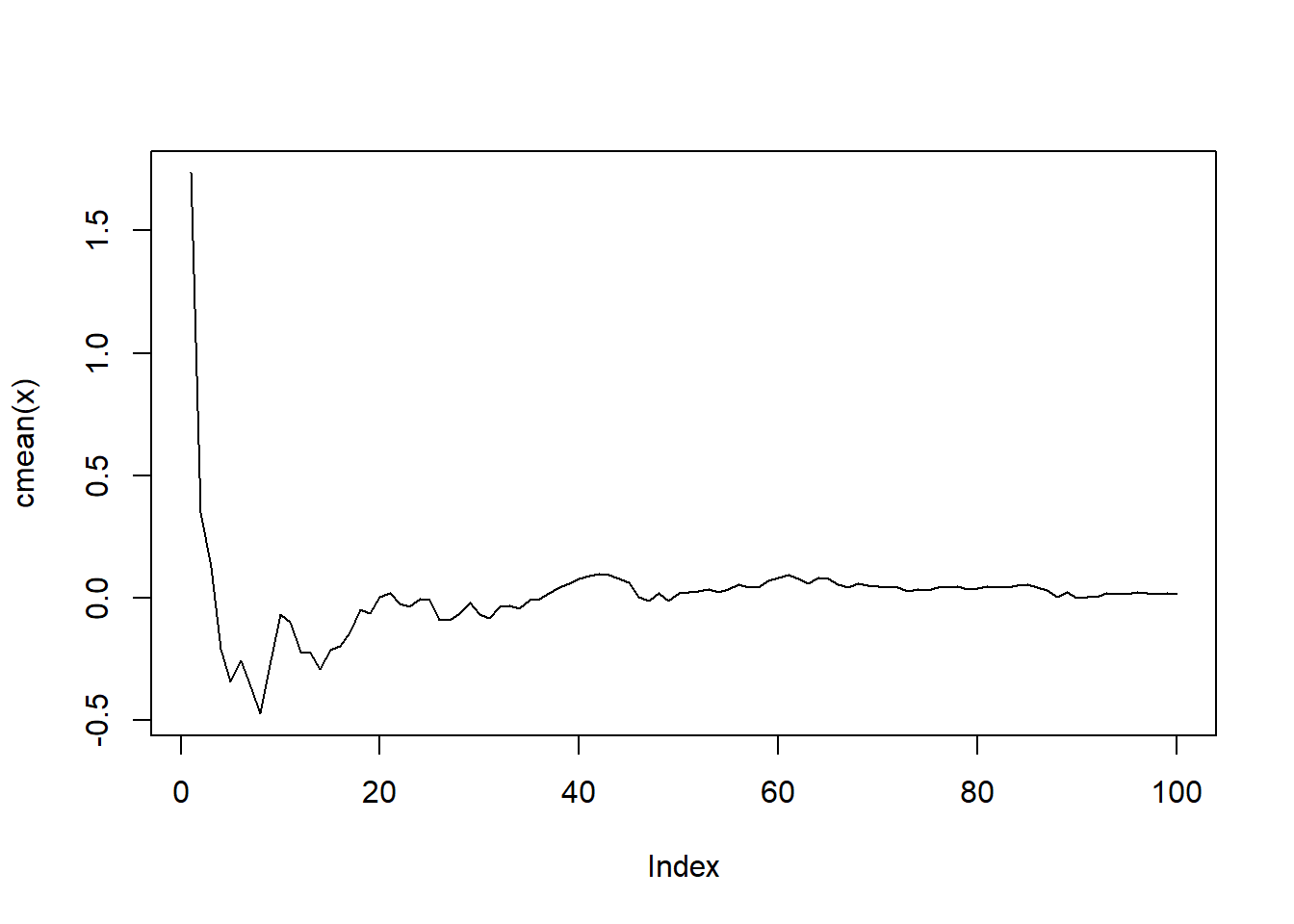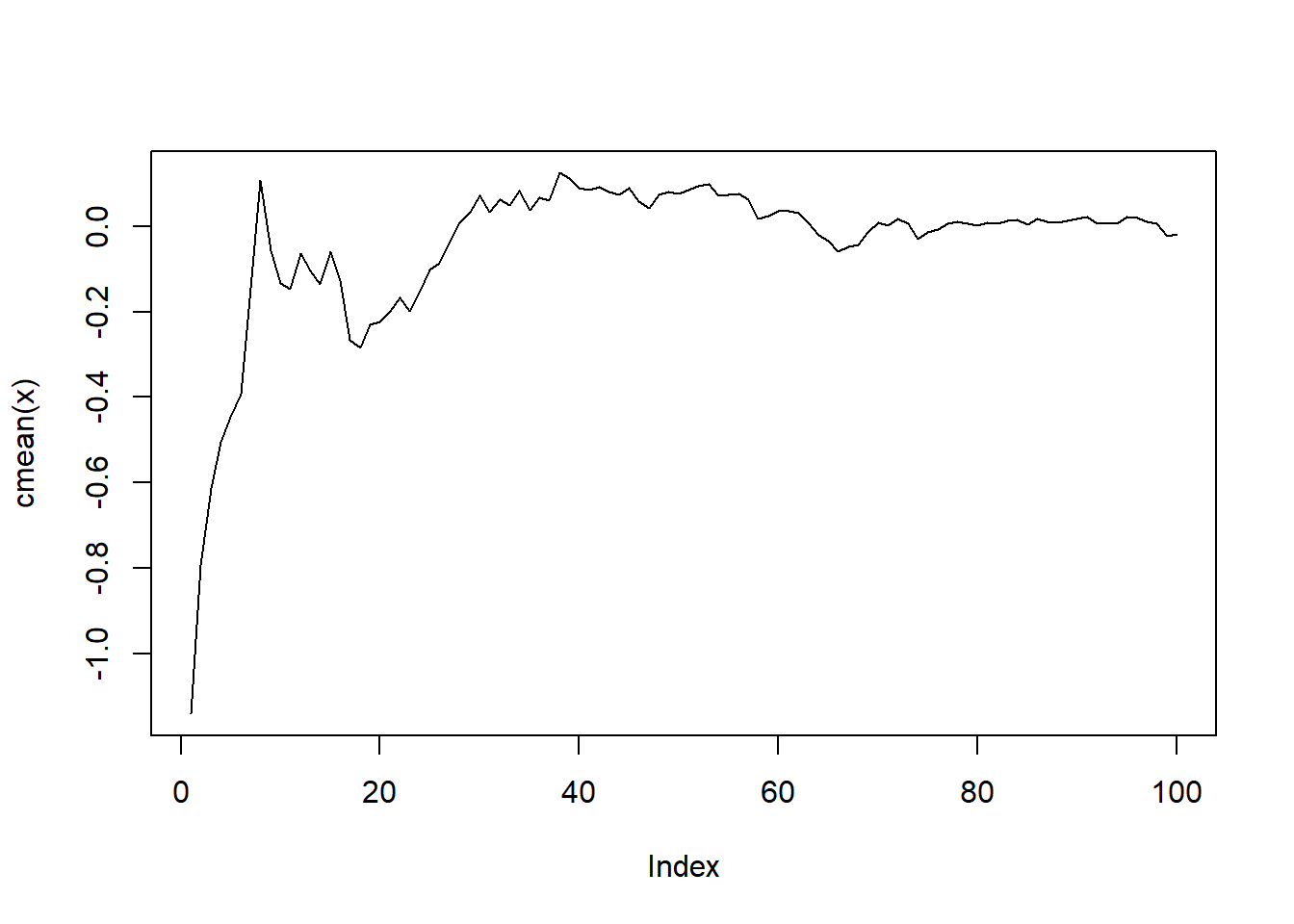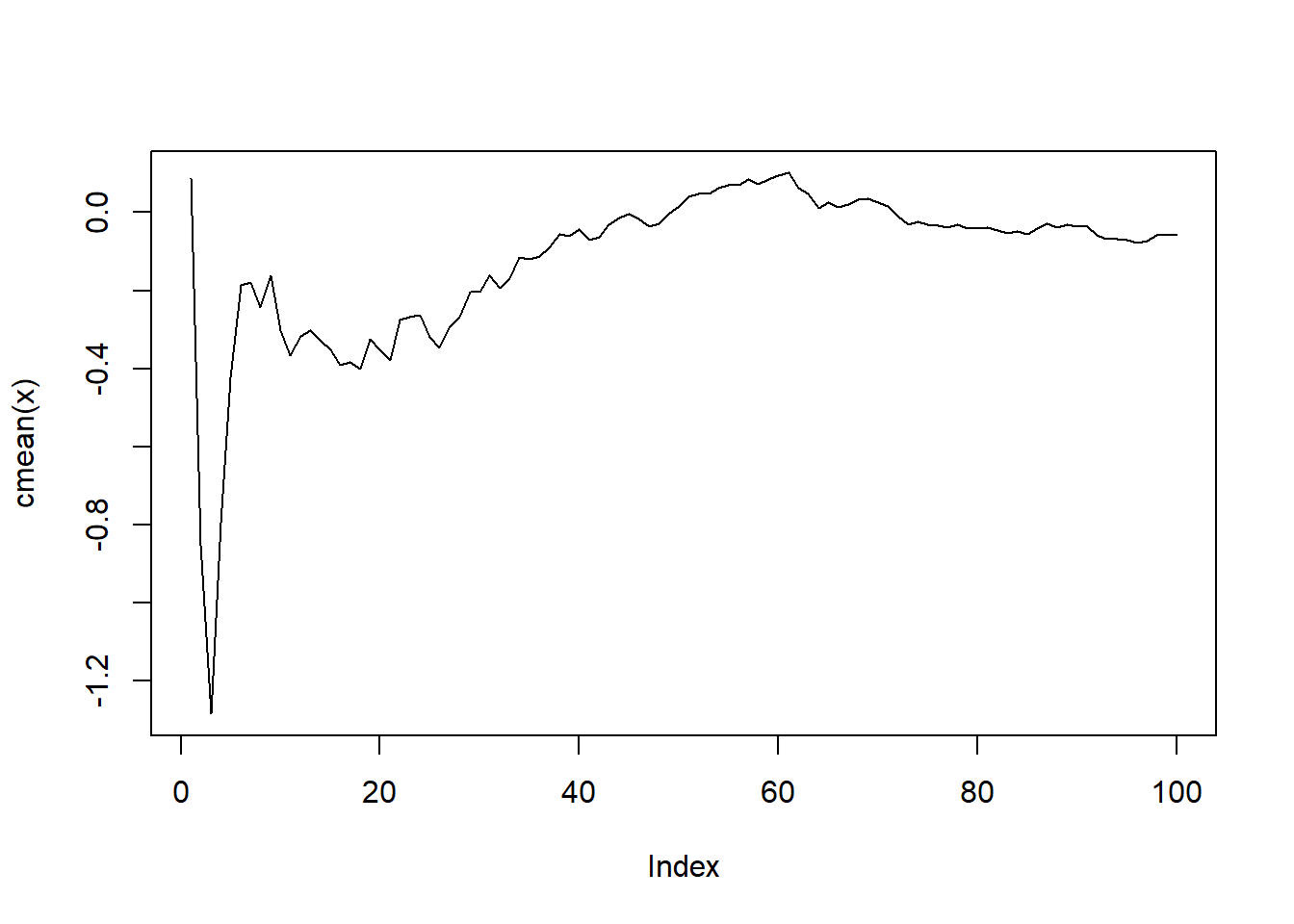``````[]
NULL

[]
NULL

[]
NULL

[]
NULL

[]
NULL``````

From here I think it is easy to see how one could do this on gruoped data as well with dplyr’s group_by().